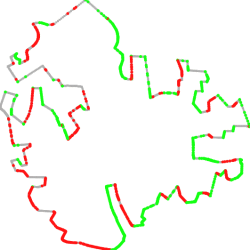# How Reliable Are Practical Point in Polygon Strategies?

Stefan Schirra

News:

 Sep 11, 2008 Polygon data updated. "Random" orthogonal polygons have been added and all real-world polygons are counterclockwise oriented now. Sep 11, 2008 Documentation updated. Sep 10, 2008 The crossing number code from Real-Time Rendering (2nd ed.) has been added to the set of strategiesThese are companion pages to "How Reliable Are Practical Point in Polygon Strategies?" (preliminary version), presented at ESA 2008. We study reliabilty, i.e., correctness and numerical robustness, of a number of strategies for testing whether a given point is contained in a given simple closed polygon. We experimentally compare several implementations, most of them publicy available on the world wide web. The selected code includes strategies from Eric Haines beautiful article on Point in Polygon Strategies published in Graphics Gems IV.

### Point-in-Polygon Tests

Our test set includes point-in-polygon code called
• crossings
• weiler
• halfplane
• barycentric
• spackman
• PNPOLY
• csg
• RTR
• CGAL
crossings, halfplane, barycentric, and spackman belong to the methods discussed in Point in Polygon Strategies. The associated code is available online. This C-code was used as is, besides that we changed function declarations and definitions from K&R-style to ANSI style for use within C++. weiler is another method discussed in an article published in Graphics Gems IV. Haines' code includes C-code for weiler as well. We refer to Point in Polygon Strategies for a discussion of these methods. PNPOLY is another implementation of a crossing number algorithm by W.R. Franklin, see PNPOLY-Point Inclusion in Polygon Test. Corresponding C-code (only a few lines of code!) is available on this page as well. csg is an implementation of a method discussed in "Practical Point-in-Polygon Tests Using CSG Representations of Polygons" by R. Walker and J. Snoeyink. The code is not publicy available and hence not included on this page. Thanks to Robert Walker for making his code available to us. Finally CGAL is template code from the Computational Geometry Algorithms Library CGAL instantiated with CGAL's simple Cartesian kernel using double precision arithmetic. RTRL is the crossing number algorithm presented in Real-Time Rendering (2nd ed.) on page 585. The authors of the book refer to Haines' code there, although that code is different. We "adapted" the code from the book.

For convenience our experiments make use of CGAL and LEDA (note that there is a LEDA free editon of LEDA available again which suffices for our purpose). LEDA is used for correctness checking and visualisation of experimental results. Thus, strictly speaking, you need both libraries in order to rerun the experiments.

### Polygon Data

Our polygon test data used in the reported experiments include real-world polygons as well as "random" polygons. The random polygons are generated by a CGAL generator using the 2-opt heuristic for a random point set. You may also create random polygons with RPG which provides additional heuristics for generating random polygons besides 2-opt. Thanks to Martin Held for making RPG availbale to us. The test polygons now also include some "random" orthogonal polygons, i.e. polygons with axis-parallel edges. Thanks to Ana Paula Tomas for making the inflate-cut code availbale to us. All polygons were scaled to the unit square.### Query Point Data

We used a number of generators to produce "difficult" query point set instances, namely query points (almost) on the edges of a polygon, query points on the "diagonals" of a polygon used by the triangle-fan based algorithms barycentric, halfplane, and spackman, points on horizontals and verticals through the vertices of a polygon, query points on the supporting lines of the edges of a polygon, and query points near the vertices of a polygon:template < class InputIterator, class OutputIterator > OutputIterator p_o_s ( InputIterator first, InputIterator beyond, OutputIterator res, int per_edge) computes per_edge query points on each edge of the polygon defined by the point range [first, beyond) and reports these points via res. Points on the edges are generated using CGAL::points_on_segment_2. If we use double precision floating-point arithmetic to compute the points, they are not necessarily exactly on the edges. For each edge both the start point and the target point are among the generated query points. template < class InputIterator, class OutputIterator > OutputIterator p_o_d ( InputIterator first, InputIterator beyond, OutputIterator res, int per_diagonal) computes per_diagonal query points on each line segment that connects the first point of the polygon with another point on the polygon and reports these points via res. Points on these "diagonals" are generated using CGAL::points_on_segment_2. If we use double precision floating-point arithmetic to compute the points, they are not necessarily exactly on the edges. For each edge both the start point and the target point are among the generated query points. template < class InputIterator, class OutputIterator > OutputIterator p_c_v ( InputIterator first, InputIterator beyond, OutputIterator res, int per_crossline) computes per_crossline query points on both the horizontal and the vertical line through each vertex, more precisely, on the intersection of these lines with the unit square, and reports these points via res. Points on these horizontal and vertical segments are generated using CGAL::points_on_segment_2. template < class InputIterator, class OutputIterator > OutputIterator p_o_l ( InputIterator first, InputIterator beyond, OutputIterator res, int per_egde) computes per_egde query points on the intersection segment of the lines supporting the polygon edges with the unit square and reports these points via res. Points on these horizontal and vertical segments are generated using CGAL::points_on_segment_2. If we use double precision floating-point arithmetic to compute the points, they are not necessarily exactly on the lines.

### Testbed Code

We use Literate Programming using LEDA's tools based on noweb. The following preliminary(!) Lweb and noweb files provide our testbed code (including query point generators, but not the point-in-polygon code itself) and documentation.
As said above, we use both CGAL and LEDA and you need noweb to extract the test file like this:

notangle -L -R'main' ReliabilityExperiments.lw > pip.C